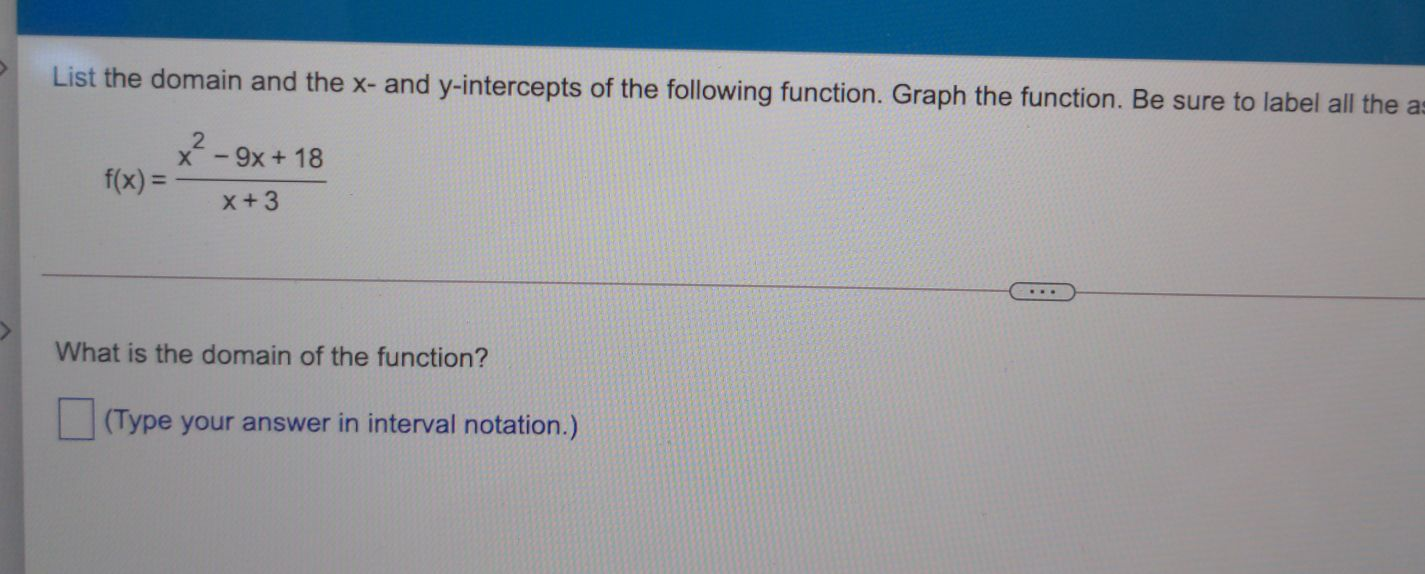### Still have math questions?

Algebra
QuestionList the domain and the $$x$$ -and $$y$$ -intercepts of the following function. Graph the function. Be sure to label all the a:

$$f ( x ) = \frac { x ^ { 2 } - 9 x + 18 } { x + 3 }$$

What is the domain of the function? (Type your answer in interval notation.)

$$Domain= (- \infty,- 3)\cup (- 3,\infty)$$